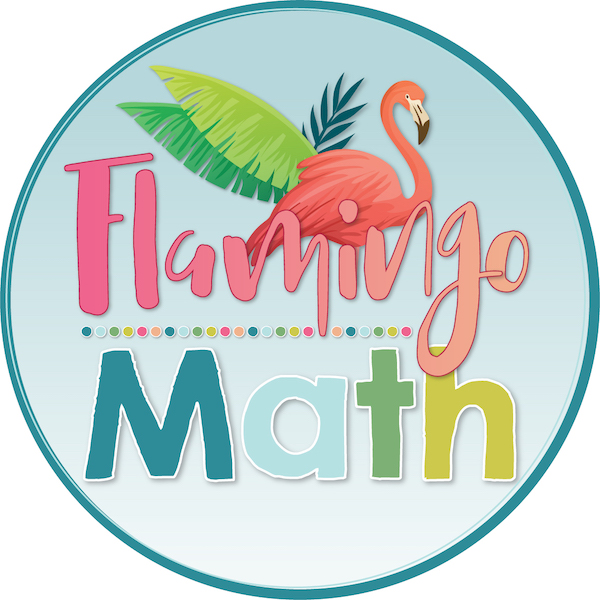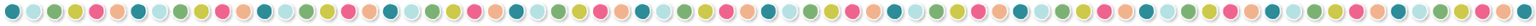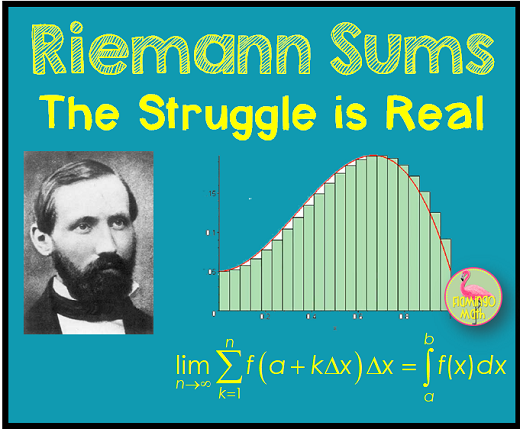# Riemann Sums – The Struggle is Real!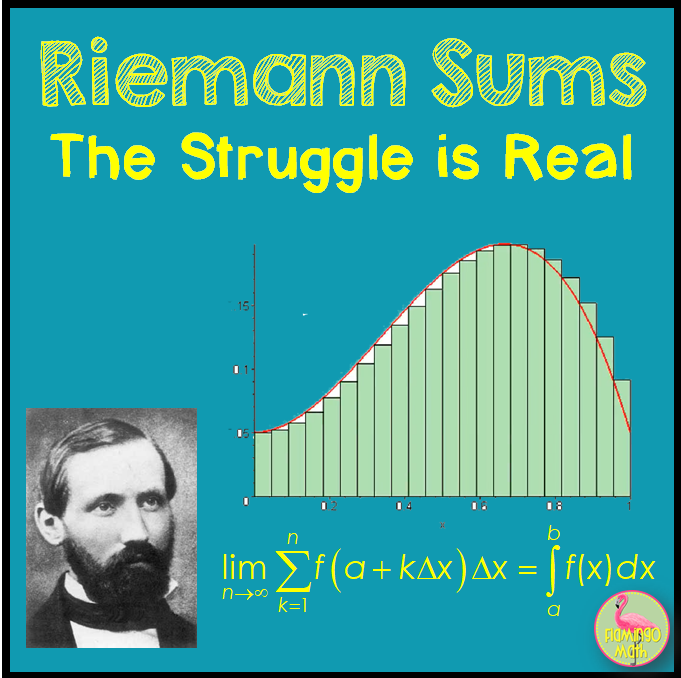## The struggle is real!

In differential calculus, we used the limit of the slopes of secant lines to define the slope of a tangent line. So, it’s only fitting that limits are also the foundation of integral calculus. After approximating area by rectangles, we discover that area can also be defined by the limit of a Riemann Sum.

Students begin by calculating area with Riemann Sum; i or k, either way, this is just a variable to indicate the individual rectangles.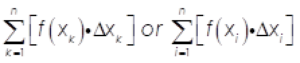Many students have experience with Summation Notation from Algebra 2 Honors or Pre-Calculus. They have seen the linearity of summation formulas: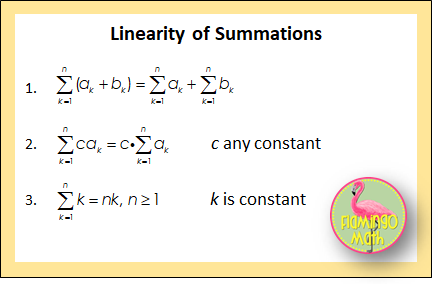Students will also prove the Power Sums Formulas, using Mathematical Induction, in Pre-Calculus: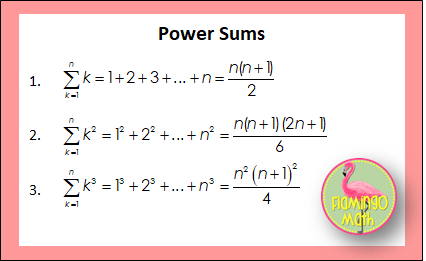While these are valuable exercises, I really want to show the concept and procedure that our Calculus students need to master. [Note: This writing will only consider the use of right-hand endpoints.] Now our students must interpret the definite integral as the limit of a Riemann sum; and, also interpret the limit of a Riemann sum as a definite integral. Let’s set the stage with the fundamental foundation in this definition: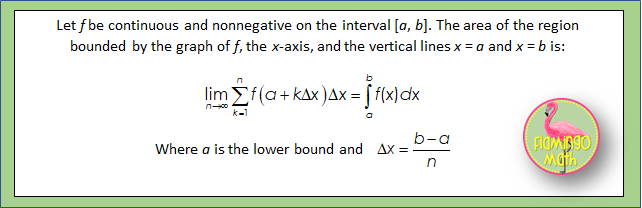Conceptually, we want our students to realize that, as the number of partition points increase (n goes to infinity) or the width of all the sub-intervals decrease (delta x goes to zero), the limit of the Riemann sum will approach the exact area between the graph and the x-axis.  You might want to assign your students the task of watching this You Tube Video lesson on Riemann Sums in Sigma Notation by Ken Schwartz.  But, come right back here, because I’ve got a surprise for you!

For example, we need our AP Calculus students to find an understanding to develop a procedure when asked to write the area as a limit, as shown in the following integral: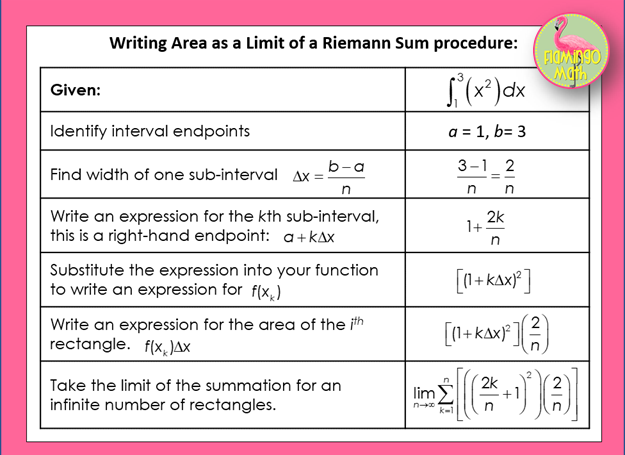Well, that’s half the battle.  Now, let’s try this in reverse.  This time you are given a limit expression and your task is to write the definite integral.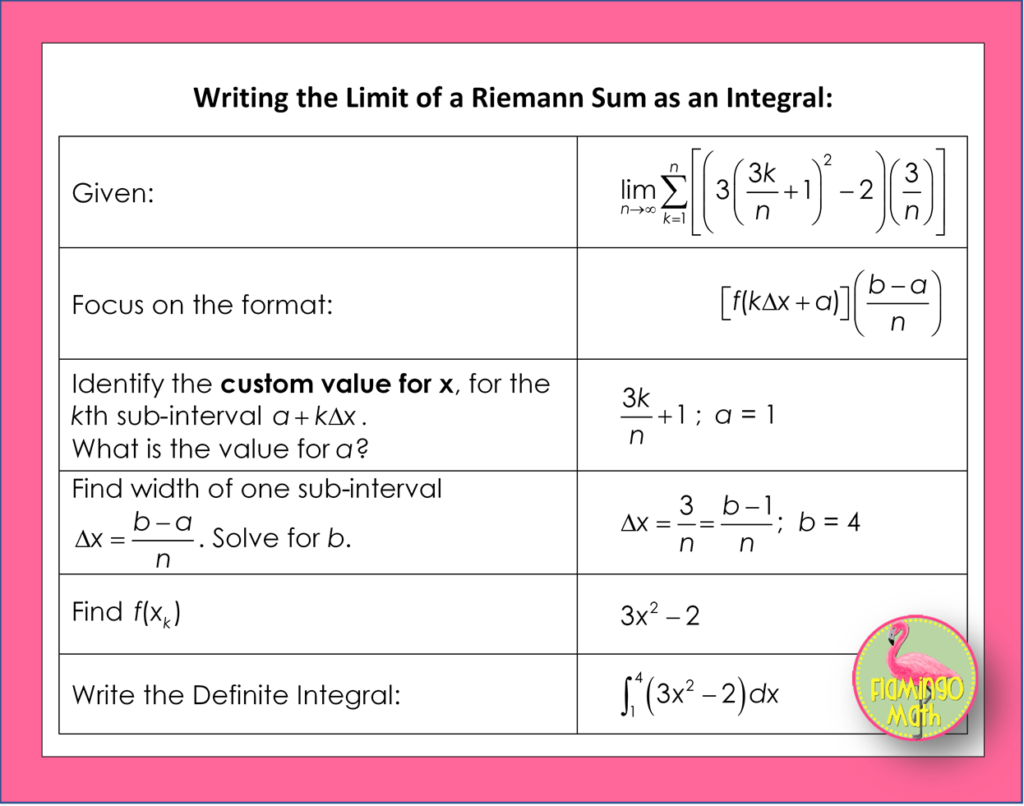I’ve made an INFOGRAPHIC for the procedure which you can grab by subscribing to our newsletter!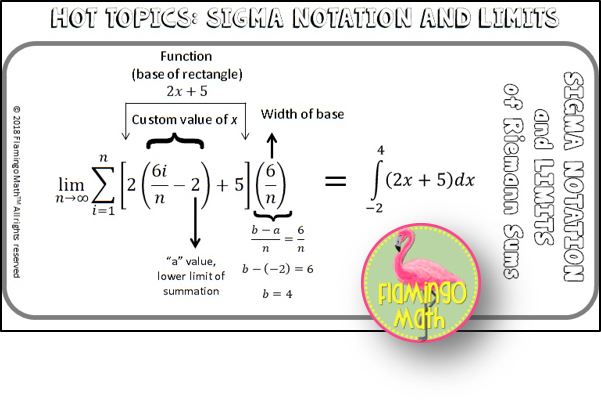### Subscribe to our mailing list!

We will send you the FREE SIGMA NOTATION INFOGRAPHIC

One more wrinkle for your consideration; suppose you have a Riemann sum in simplified form? Can you “unsimplify” to find the definite integral?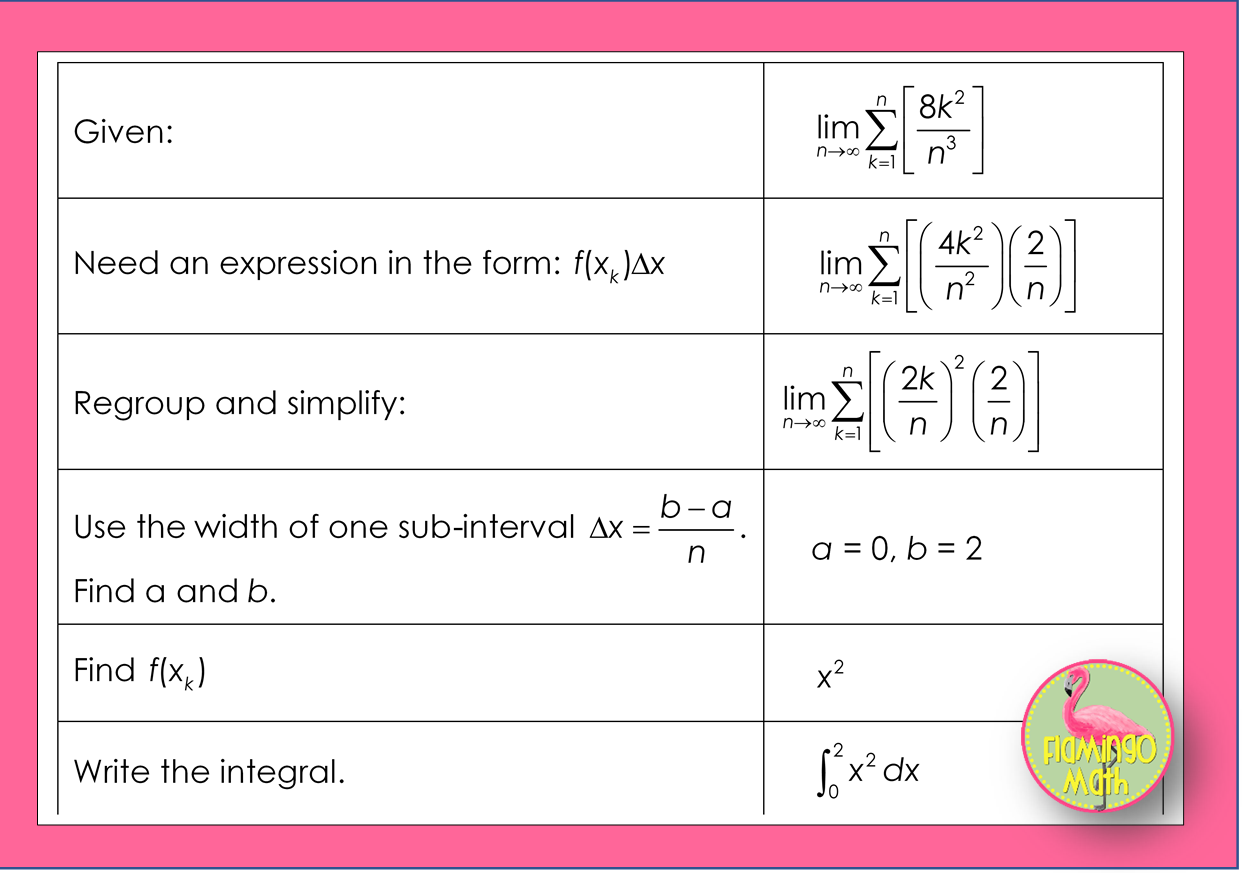Do you need a complete lesson, extra practice, or task cards, on this topic for you and your students?  If so, be sure to check out

#### HOT TOPICS: Sigma Notation and Limits of Riemann Sums

What other lessons give you teaching challenges? Leave me a comment below and I’ll be happy to blog about that! Be sure to grab the FREE INFOGRAPHIC!## You may also like...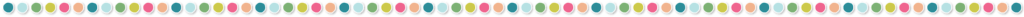### 2 Responses

1.Sue Popelka says:

i subscribed to the newsletter but I did not receive the free infographic on Riemann Sums advertised.

1.flamingomath_vjbfdj says:

Hey Sue,
I will check that out for you. I’m new at this. If you send me an email to jean@dreamsites.io I will send it to you personally.
Sorry for the mix up.
Jean

This site uses Akismet to reduce spam. Learn how your comment data is processed.

## Let's connect